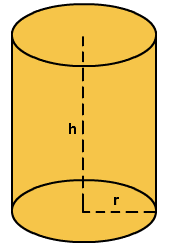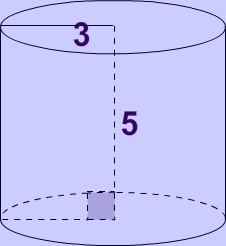How to Calculate and Solve for the Volume, Radius and Height of a Cylinder | Nickzom CalculatorThe image above represents a cylinder.
To compute the volume of a cylinder requires two essential parameters which are the radius and height of the cylinder.

The formula for computing the volume of a cylinder is:

V = πr2h

Where:
V = Volume of a cylinder
r = radius of the cylinder
h = height of the cylinder

Let’s solve an example
Find the volume of a cylinder with a radius of 3 cm and a height of 5 cm.

This implies that:
r = radius of the cylinder = 3
h = height of the cylinder = 5

V = πr2h
V = 3.142 x 32 x 5
V = 141.39

Therefore, the volume of the cylinder is 141.39 cm3.

Calculating the Height of a cylinder when Volume and Radius is Given

The formula is h = V / πr2

Where;
V = Volume of a cylinder
r =  radius of the cylinder
h = height of the cylinder

Let’s solve an example:
Find the height of a cylinder with a volume of 300 cm3 and a radius of 3 cm

This implies that;
V = Volume of the cylinder = 300 cm3
r  = radius of the cylinder = 3 cm

h = V / πr2
h = 300 / 3.142(3)2
h = 300 / 28.278
h = 10.61
Therefore, the height of the cylinder is 10.61 cm.

Calculating the Radius of a cylinder when Volume and Height is Given

The formula is r = √(V / πh)

Where;
V = Volume of a cylinder
r =  radius of the cylinder
h = height of the cylinder

Let’s solve an example:
Find the radius of a cylinder with a volume of 200 cm3 and a height of 5 cm

This implies that;
V = Volume of the cylinder = 200 cm3
h  = height of the cylinder = 5 cm

r = √(V / πh)
r = √(200 / 3.142(5))
r = √(200 / 15.71)
r = √12.73
r = 3.57

Therefore, the radius of the cylinder is 3.57 cm.

How to Calculate the Area, Radius and Height of a Cylinder | Nickzom CalculatorThe image above represents a cylinder.
To compute the area of a cylinder requires two essential parameters which are the radius and height of the cylinder.

The formula for computing the area of a cylinder is:

A = 2πrh + 2πr2

Where:
A = Area of a cylinder
r = radius of the cylinder
h = height of the cylinder

Let’s solve an example
Find the area of a cylinder with a radius of 3 cm and a height of 5 cm.

This implies that:
r = radius of the cylinder = 3
h = height of the cylinder = 5

A = 2πrh + 2πr2
A = 2(3.142) x 3 x 5 + 2(3.142) x 32
A = 150.82

Therefore, the area of the cylinder is 150.82 cm2.

Calculating the Height of a cylinder when Area and Radius is Given

The formula is h =  A/ 2πr – r

Where;
A = Area of a cylinder
r =  radius of the cylinder
h = height of the cylinder

Let’s solve an example:
Find the height of a cylinder with an area of 600 cm² and a radius of 5 cm

This implies that;
A = Area of the cylinder = 600 cm²
r  = radius of the cylinder = 5 cm

h = A / 2πr – r
h = 600 / 2(3.142)(5) – 5
h = 600 / 31.42 – 5
h = 19.10 – 5
h = 14.10

Therefore, the height of the cylinder is 14.10 cm.